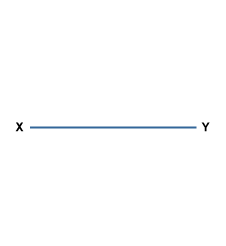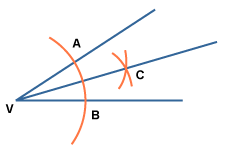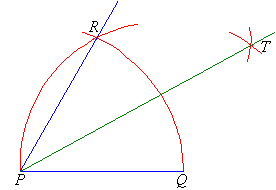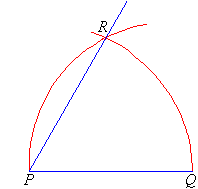Geometrical Construction

Using ruler and compasses: Remember the following when making geometrical constructions.

1. Use a hard pencil with a sharp point. This gives thin lines which are more accurate.

2. Check that your ruler has good straight edge. A damaged ruler is useless for construction work.

3. Check that your compasses are not too loose. Tighten loose compasses with a small screw driver.

4. All construction lines must be seen. Do not rub out anything which leads to the final result.

5. Always take great care, especially when drawing a line through a point.

6. Where possible, arrange that the angles of intersection between lines and arcs are about 900.

Perpendicular bisector of a line segment

The locus of a point which moves so that it is an equal distance from two points, A and B, is the perpendicular bisector of the line joining A and B.

Perpendicular means at right angles to.

Bisector means cuts in half.

To construct this locus, you do the following (try this yourself on a piece of paper):Draw the line segment XY.Put your compass on X and set it to be over half way along the line. Draw an arc.Without adjusting your compass put it on Y and draw another arc.Label these points A and B.Draw a straight line through A and B.The point M where the lines cross is the midpoint of XY. And AB is perpendicular to XY.Bisecting an angle

V is the vertex of the angle we want to bisect.Place your compass on V and draw an arc that crosses both sides of the angle.Label the crossing points A and B.Place your compass on A and draw an arc between the two sides of the angle.Without adjusting your compass place it on B and draw another arc that cuts the one you just drew. Label the point where they cross C.Draw a straight line through V and C.The line VC bisects the angle. Angles AVC and BVC are equal.Constructing a 900 Angle

We can construct a 90º angle either by bisecting a straight angle or using the following steps.

Step 1:  Draw the arm PA.
Step 2:  Place the point of the compass at P and draw an arc that cuts the arm at Q.
Step 3:  Place the point of the compass at Q and draw an arc of radius PQ that cuts the arc drawn in Step 2 at R.
Step 4:  With the point of the compass at R, draw an arc of radius PQ to cut the arc drawn in Step 2 at S.
Step 5:  With the point of the compass still at R, draw another arc of radius PQ near T as shown.
Step 6:  With the point of the compass at S, draw an arc of radius PQ to cut the arc drawn in step 5 at T.
Step 7:  Join T to P. The angle APT is 90º.Constructing a 300 Angle

We know that: ½ of 600 = 300

So, to construct an angle of 30º, first construct a 60º angle and then bisect it. Often, we apply the following steps.

Step 1:  Draw the arm PQ.
Step 2:  Place the point of the compass at P and draw an arc that passes through Q.
Step 3:  Place the point of the compass at Q and draw an arc that cuts the arc drawn in Step 2 at R.
Step 4:  With the point of the compass still at Q, draw an arc near T as shown.
Step 5:  With the point of the compass at R, draw an arc to cut the arc drawn in Step 4 at T.
Step 6:  Join T to P.  The angle QPT is 30º.Constructing a 600 Angle

We know that the angles in an equilateral triangle are all 60º in size.  This suggests that to construct a 60º angle we need to construct an equilateral triangle as described below.

Step 1:  Draw the arm PQ.
Step 2:  Place the point of the compass at P and draw an arc that passes through Q.
Step 3:  Place the point of the compass at Q and draw an arc that passes through P.  Let this arc cut the arc drawn in Step 2 at R.

Step 4: Join P to R. The angle QPR is 600, as the ∆PQR is an equilateral triangle.Try your understanding regarding the explanations above over and over again.